# Calculating Bend Allowance, Bend Deduction, and K-FactorArticle by Saeed Mojarad (CSWE) updated June 6, 2017

###### Article

In my previous post I talked about K-Factor, Bend Allowance and Bend Deduction and what they mean in sheet metal design. Now let’s see how we can obtain these values for a specific sheet.

As I mentioned in my last post you need to do some tests to calculate these values for a specific sheet. These tests include bending some samples and then do some measurements and calculations.

Consider a sheet with a 20 mm thickness and a length of 300 mm as shown in Figure 1. We are going to review three bending scenarios with three different bending angles; 60, 90 and 120, and we will calculate K-Factor, Bend Allowance and Bend Deduction for them. The bending tool has a radius of 30 mm which means that our Inside Bend Radius (R) is 30 mm. Let’s start with 90 degrees bend which is the most simple scenario.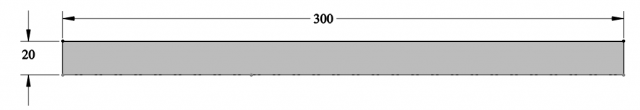Figure 1: Flat sheet

### 90 Degrees Bend Angle

Figure 2 illustrates the sheet that is bent with the bend angle of 90 degrees. We will start by calculating the Bend Allowance. From there we can calculate the K-Factor and the Bend Deduction. After bending the sheet we need to do some measurements as shown in Figure 2.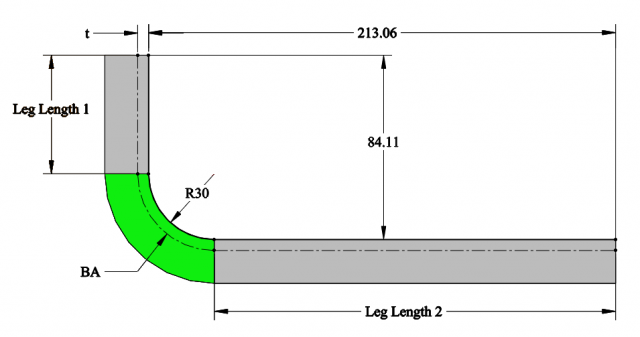Figure 2: 90 degrees bend

We can calculate the Leg Length 1 and 2 as follows: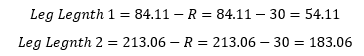At the neutral axis we have: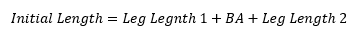In this formula the initial length is 300 mm. By replacing Initial Length, Leg Length 1 and 2 in the above equation we can calculate the Bend Allowance as follows:We know that BA is the length of the arc on the neutral axis. The length of the arc for this scenario can be calculated as: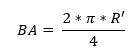Where R’ is the radius of the arc on the neutral axis. By inserting the Bend Allowance value in the above equation we reach to: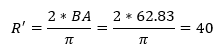Now if we subtract R from R’ we can find the distance of the neutral axis (t) from the inner face: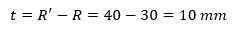From the K-Factor equation we have:# Need Help with your Sheet Metal Setup?

Our SOLIDWORKS Experts can setup your environment so that your team uses a comprehensive set of templates, tables, and library of forming tools

### Bending Angles Less Than 90 degrees

For our second scenario we are going to discuss the calculations for bending angles less than 90 degrees. As an example we are going to use 60 degrees as our bending angle. Again we have to do some measurements as shown in Figure 3. Then we have to calculate Leg Length 1 and Leg Length 2.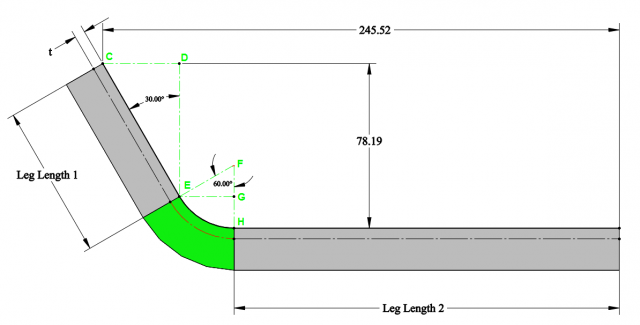Figure 3: 60 degrees bend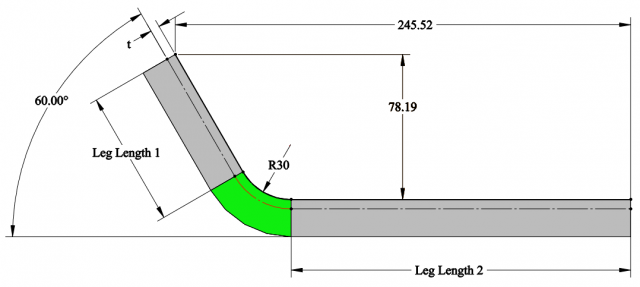Let’s start by calculating Leg Length 1. From figure 3 we know that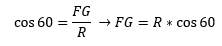Where R is the Inside bend radius which is equal to 30 mm in this example. We can calculate Leg Length 1 through a few simple equations as follow: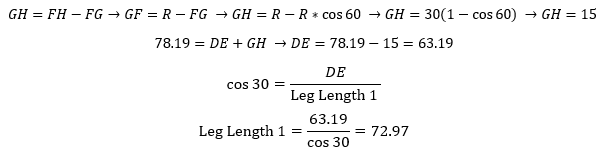Now let’s calculate Leg Length 2: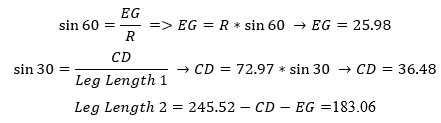Now that we have both Leg Length 1 and 2 we can use the following equation again to calculate the Bend Allowance: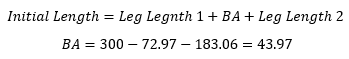To calculate R’ which is the radius of the arc on the neutral axis we can use the following equation: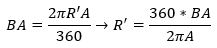A is the bending angle in the above equation so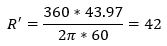To calculate the neutral axis distance from the inner face (t) we can subtract inside bend radius from R’: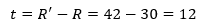And by having t and the sheet thickness (T) we can calculate the K-Factor as follow: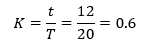### Bending Angles Greater Than 90 degrees

Like previous scenarios let’s start by calculating Leg Length 1.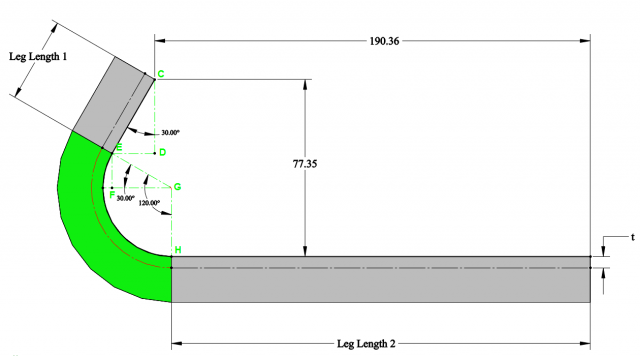Figure 4: 120 degrees bend

Based on Figure 4 we have: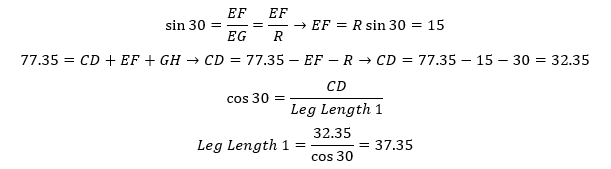Next we calculate Leg Length 2: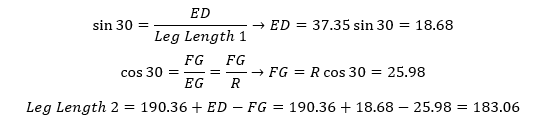Now we can calculate the Bending Allowance: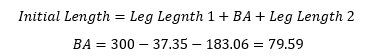By having BA we can now calculate K-Factor: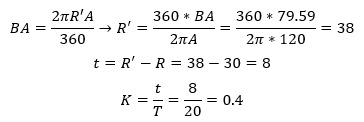### Bend Deduction Calculation

As explained in my first post the Bend Deduction can be calculated using the following equation: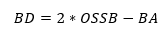Where OSSB is the outside setback. OSSB is defined as illustrated in figure 5 for different bending angles and can be calculated using the equation below: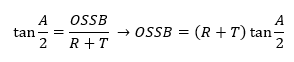Where A is the bending angle,T is the sheet thickness and R is the bending radius.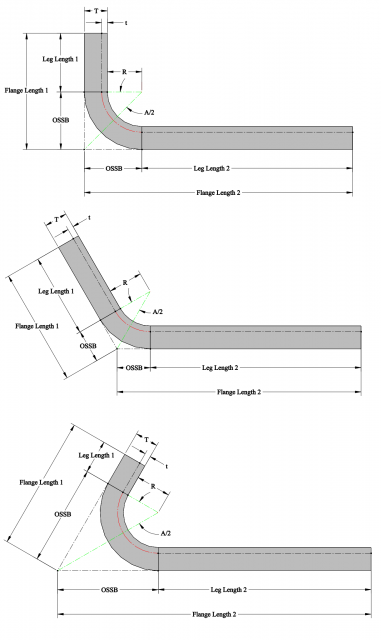Figure 5: outside setback (OSSB) in different bending angles

In the next post we are going to talk about bend and gauge tables in SOLIDWORKS and how we can use the numbers we calculated here to make our own bend and gauge tables.

#### Get Certified SOLIDWORKS Services from Javelin